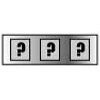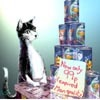#### You may also like### Summing Consecutive Numbers

15 = 7 + 8 and 10 = 1 + 2 + 3 + 4. Can you say which numbers can be expressed as the sum of two or more consecutive integers?### Forgotten Number

I have forgotten the number of the combination of the lock on my briefcase. I did have a method for remembering it...### Human Food

Sam displays cans in 3 triangular stacks. With the same number he could make one large triangular stack or stack them all in a square based pyramid. How many cans are there how were they arranged?

# Handshakes

##### Age 11 to 14Challenge Level

Seven mathematicians met up one week.

The first mathematician shook hands with all the others.
The second one shook hands with all the others apart from the first one (since they had already shaken hands).
The third one shook hands with all the others apart from the first and the second mathematicians, and so on, until everyone had shaken hands with everyone else.

How many handshakes were there altogether?The next week, eight mathematicians met. How many handshakes took place this time?
The following week, there were nine mathematicians...

Sam is trying to work out how many handshakes there would be if 20 mathematicians met. He says that since each mathematician shakes hands 19 times, there must be $20 \times 19$ handshakes altogether. Helen disagrees; she worked out $19 + 18 + 17 + ... + 2 + 1$ and got a different answer. What is wrong with Sam's reasoning? How should he modify his method?

One day, 161 mathematicians met. How many handshakes took place this time?

Can you describe a quick way of working out the number of handshakes for any size of mathematical gathering?

Could there be exactly 4851 handshakes at a gathering where everyone shakes hands? How many mathematicians would there be?

What about the following numbers of handshakes?
• 6214
• 3655
• 7626
• 8656

You may wish to try the problems Picturing Triangle Numbers and Mystic Rose. Can you see why we chose to publish these three problems together?

You may also be interested in reading the article Clever Carl, the story of a young mathematician who came up with an efficient method for adding lots of consecutive numbers.